# Vector quantities

## Introduction

The quantities available for post-processing can be scalar or vector quantities.

This section deals with vector quantities and recalls some definitions: complex notation, elliptical representation…

## Vector quantities (complex notation as rotating vectors)

Each component of a vector quantity can be written as a sinusoidal quantity: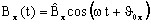; …

or, in complex notation: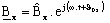; …; …

where:

•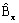is the modulus of the complex component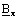•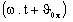is the argument (or the phase) of the complex component•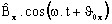is the real part of the complex component•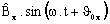is the imaginary part of the complex component## Elliptical representation in 2D

In general case, the vector quantities are varying in function of time on ellipses.

For each point, the magnetic flux density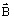can be written: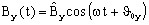These are the parametric equations of an ellipse.The two components of the magnetic flux density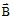can be expressed depending on the ellipse characteristics, as follows:

•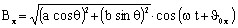, where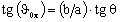•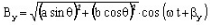where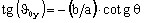a is called “½ major axis” and b is called “½ minor axis